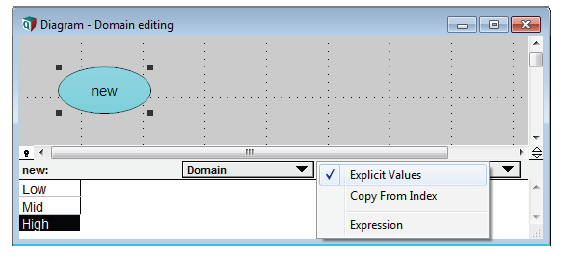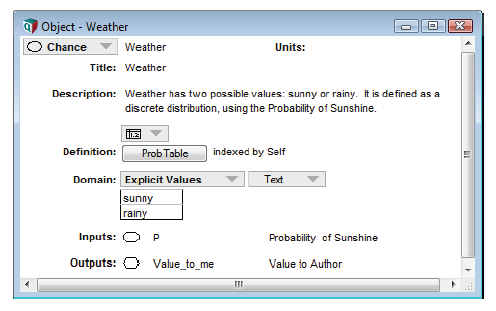# The domain attribute and discrete variables

The domain attribute specifies the set of possible values for a variable. You rarely need to view or change a domain attribute explicitly. The most common reason to set the domain is for a variable defined as a custom discrete distribution, especially ProbTable. You can do this by editing it directly as an index in the probtable view, so you can usually ignore the information below. The rare case you need it is to specify a distribution as discrete, when Analytica would not otherwise figure it out — because it has non-integer numerical value.

By default, the domain type is Automatic, meaning Analytica figures it out when it needs to. Usually, this is obvious (see previous section). For a discrete quantity, the domain can be a list of numbers or a list of labels. If the domain is continuous, it means that any number is valid.

Editing the domain: You can view and edit the domain like any other attribute of a variable, in the Attribute panel:

1. Select the variable.
2. Open the Attribute panel, and select Domain from the Attribute dropdown menu.
3. Select the domain type from the popup menu.The domain type:

Automatic The default, meaning Analytica should figure it out. Each cell of the result is a real number. Lower and upper bounds may be optionally specified. There is also a semi-continuous variation in which the value must be between the indicated bounds or it may be zero. The semi-continuous variation must be entered as an Expression, e.g., Continuous(100K, 1M, orZero: True). When defined as continuous, uncertain variables are treated as continuous, whereas the other types are discrete. Each cell of the result in an integer, with lower and upper bounds optionally specified. Uncertain results are graphed as discrete numeric.The Optimizer searches for an integer solution. (Optimizer edition only) All scalar decision variables belonging to the same group must have a value between 1 and n, where n is the number of cells belonging to the same group, and all the values of each must be different. Each cell of the result is 0 (False) or 1 (True) valued. Discrete but its values are unspecified. Influences how uncertain quantities are treated and in some cases, how graph axes are scaled by default. You specify a list of possible values. Within this option, you can also select whether these possible values are to be limited to be textual or numeric. You enter the name of an index variable, to use its values as the domain, or another variable to copy its domain values. An arbitrary expression that computes the domain type, ranges and possible values. The expression should return with a call to one of the functions Continuous, Integer, GroupedInteger, or Discrete, or it can return a list of values. The expression can contain arbitrary logic and can use values from other variables in the model.

Domain in the Object window: You can also view and edit the domain attribute in the Object window if you set it to do so in the Attributes dialog (see Managing attributes)Tip

The domain of a discrete variable should include all its possible values. If not, its probability mass function might sum to less than 1.